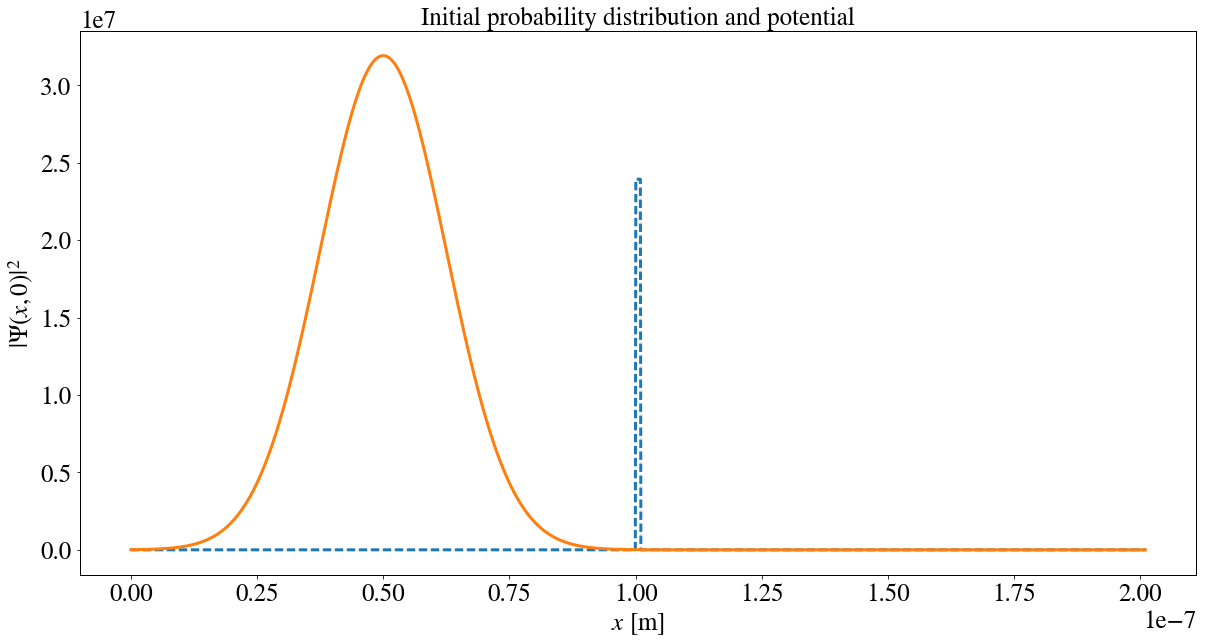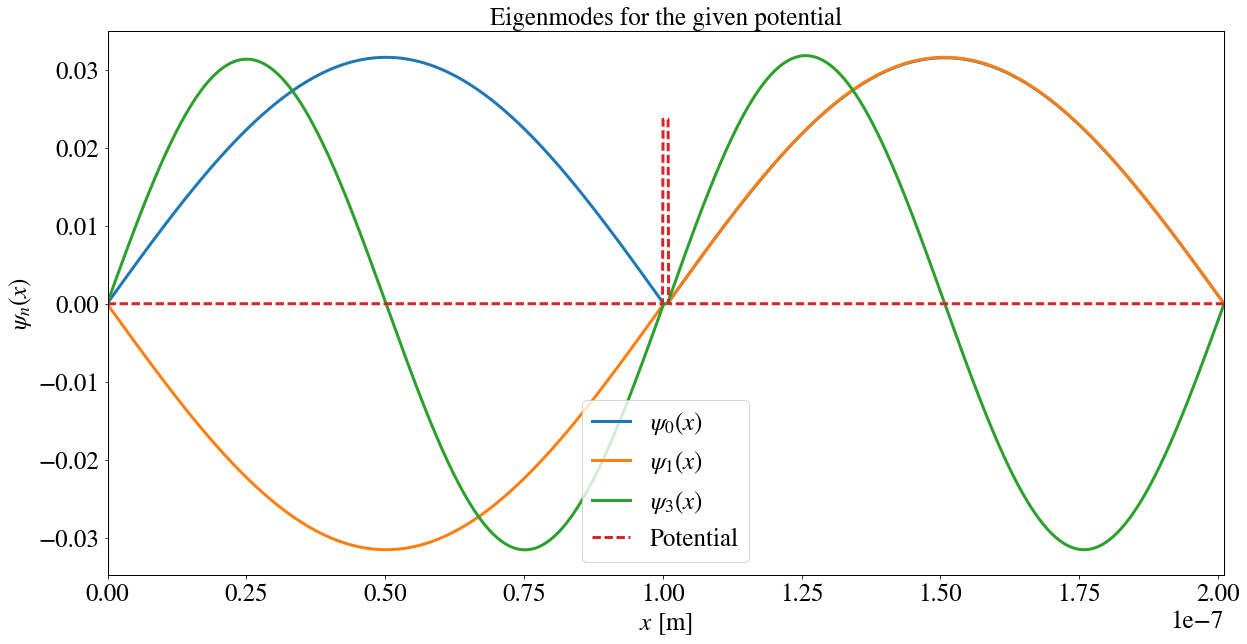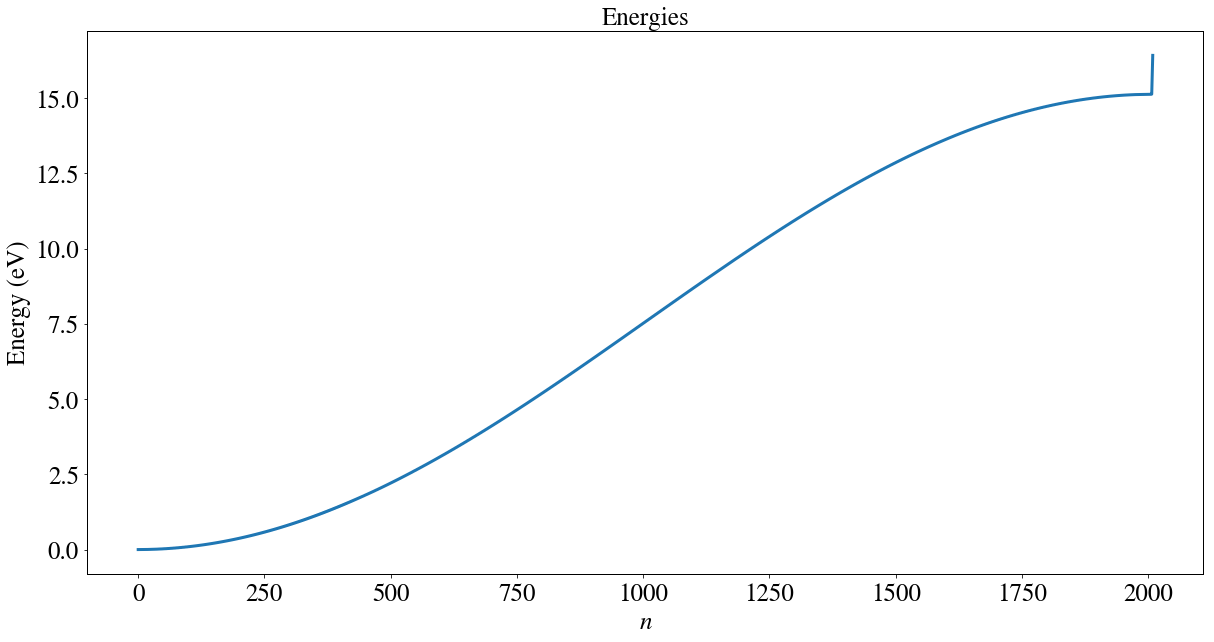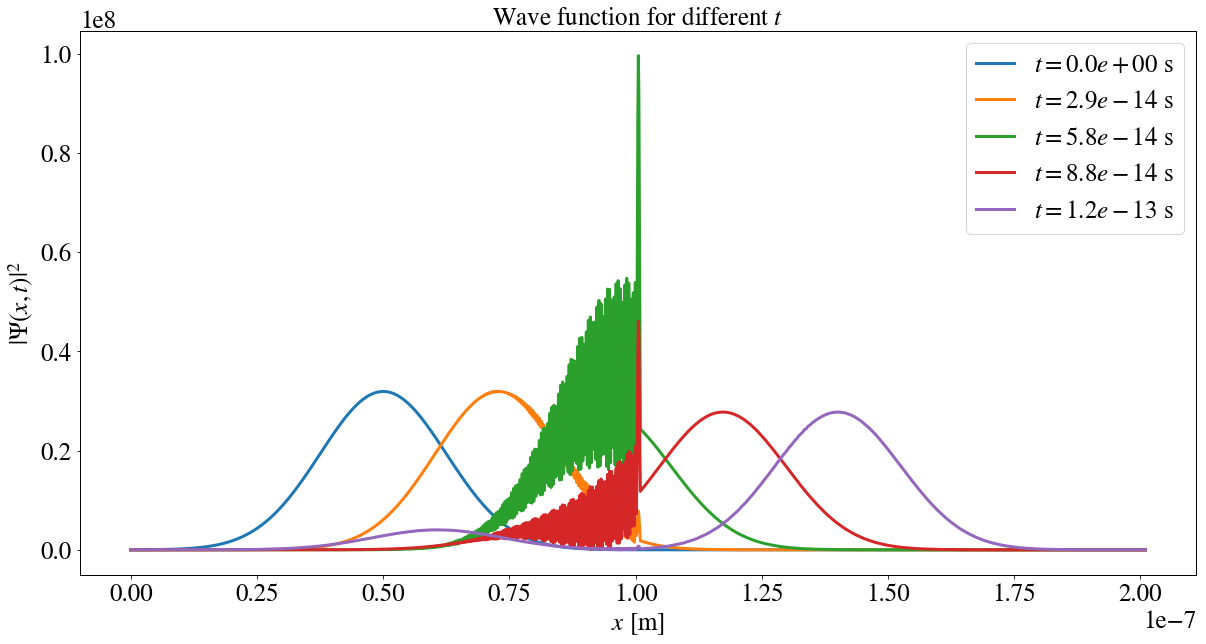# Solving the Schrödinger equation numerically by expansion in eigenstates¶

## Examples – Quantum Mechanics¶

Last edited: January 20th 2019

## Introduction¶

In this notebook we will be solving the one-dimensional Schrödinger equation,

$$i\hbar\frac{\partial\Psi(x, t)}{\partial t} = -\frac{\hbar^2}{2m}\frac{\partial^2 \Psi( x, t)}{\partial x^2}+V(x)\Psi( x, t)$$

numerically for an arbitrary initial condition $\Psi(x, 0)$. The eigenstates $\psi_n(x)$ and the eigenenergies $E_n$ of the system are found by solving the time-independent Schrödinger equation

$$-\frac{\hbar^2}{2m}\frac{\partial^2 \psi_n(x)}{\partial x^2}+V(x)\psi_n(x) = E_n\psi_n(x),$$

and normalizing the result. The inital condition $\Psi(x, 0)$ is expanded in terms of $\psi_n(x)$:

$$\Psi(x,0) = \sum_{i}\alpha_i\psi_i(x).$$

In turn, the solution at time $t$, $\Psi(x, t)$, is given by

$$\Psi(x, t) = \sum_n\alpha_n\psi_n(x)\exp\left(-i\frac{E_n}{\hbar}t\right).$$

As an example, we will be propagating an electron given by a gaussian wave packet towards a potential barrier. A similar example is studied in our notebook on One-Dimensional Wave Packet Propagation, but with a quite different approach.

The numerical scheme that is used is developed and explained in detail in the appendix at the end of this notebook. The reader is adviced to read through this before reviewing the notebook.

We start by importing packages, setting common figure parameters and defining physical parameters.

In :
import matplotlib.pyplot as plt
from scipy.linalg.lapack import ssbevd
import numpy as np
from matplotlib import animation

newparams = {'axes.labelsize': 25, 'axes.linewidth': 1, 'savefig.dpi': 200,
'lines.linewidth': 3, 'figure.figsize': (20, 10),
'ytick.labelsize': 25, 'xtick.labelsize': 25,
'figure.titlesize': 25,
'legend.fontsize': 25, 'legend.frameon': True,
'legend.handlelength': 1.5, 'axes.titlesize': 25,
'mathtext.fontset': 'stix',
'font.family': 'STIXGeneral'}
plt.rcParams.update(newparams)

hbar = 1.05E-34  # J⋅s. Reduced Plank's constant
m = 9.11E-31     # kg.  Electron mass


## Potential¶

As mentioned in the introduction, we will be propagating an electron towards a potential barrier in one dimension. We will be considering a domain $x\in[0,L]$. Let us use $\Delta x = 1\text Å$, which is a typical diameter of an atom. In turn, the width of the barrier is being decided by the number of discretization points it consists of. We want each side of the potential barrier to be large, so that the electron is not influenced by the barrier or the edges at $t=0$. We choose $N=10$ discretization points for the barrier, and 50 times that for each of the sides. The barrier has a height $V_0=1.5\cdot 1.6\cdot 10^{-19}J = 1.5\text{eV}$.

Play around with other parameters and potential barriers. The code in this notebook works even for arbitrary potentials!

In :
V0 = 1.5*1.6E-19 # J. Potential height
dx = 1e-10       # m. Discretization step
N = 10           # #. Number of discretization points in the barrier
N_sides = 100*N  # #. Number of discretization points on each side of the barrier

Ntot = N + 2*N_sides # Total number of discretization points
x = np.linspace(0, dx*Ntot, Ntot) # x-axis
# Potential
V = np.array(*N_sides + [V0]*N + *N_sides)


## Wave packet¶

We will be representing the initial electron as a gaussian wavepacket, $$\Psi(x,0)=C\exp\left(-\frac{(x-x_0)^2}{4\sigma^2}+i\frac{p_0x}{\hbar}\right),$$ where $p_0=\sqrt{2mE_0}$ is the momentum of the wave packet, $E_0$ the energy of the electron, $x_0$ is the initial expectation value, and $\sigma$ is some parameter specifying the width of the wave packet.

It will not be unreasonable to choose $E_0\sim V_0$. As we will see, this will give a good visualization of transmission and reflection. We start by choosing an energy a bit higher than the potential height, $E_0=1.39V_0$. $x_0$ to be in the middle of the left part of the domain and $\sigma$ (one standard deviation) to 1/8 of the left part. Play around with different parameters!

In :
x0 = 0.5*dx*N_sides
E0 = 1.390*V0
k0 = np.sqrt(2.0*m*E0)/hbar
sigma = dx*N_sides/8.
A = (2*np.pi*sigma**2)**(-0.25)
Psi_0 = A * np.exp(-(x-x0)**2/(4*sigma**2)) * np.exp(1j*k0*x)
# Check if the wave function is normalized
print("Normalization:", dx*np.sum(np.abs(Psi_0)**2))

Normalization: 0.999471364542


We now visualize the initial wave packet and the potential (with a suitable scaling).

In :
plt.plot(x, .75*V*np.max(np.abs(Psi_0)**2)/max(1e-30,np.max(V)), '--')
plt.plot(x, np.abs(Psi_0)**2)
plt.title('Initial probability distribution and potential')
plt.xlabel('$x$ [m]')
plt.ylabel('$|\Psi(x,0)|^2$')
plt.show()## Solving the eigenvalue problem (Schrödinger equation)¶

Now that all the parameters are settled, we can finally solve the Schrödinger equation. This is done by solving an eigenvalue problem. We are using a real symmetric band matrix solver (You could of course also use numpy.linalg.eigh, but this requires the initialization of the whole matrix, mostly consisting of zeros). We thus need to initialize the diagonal and the sub- and superdiagonal. This is explained in detail in the appendices.

Note that this is the most computationally demanding part of these computations.

In :
diag = hbar**2/(m*dx**2) + V # Diagonal
sup_diag = np.ones(Ntot)*(-hbar**2/(2*m*dx**2)) # Superdiagonal

In :
E, psi_n, _ = ssbevd([sup_diag, diag]) # Call solver


Let us visualize some of the eigenstates and eigenenergies!

In :
for i in [0, 1, 3]:
plt.plot(x, psi_n[:,i], label=r"$\psi_{%.0f}(x)$"%(i))
plt.plot(x, .75*V*np.max(psi_n)/max(1e-30,np.max(V)), '--', label="Potential")
plt.title("Eigenmodes for the given potential")
plt.xlabel("$x$ [m]")
plt.ylabel("$\psi_n(x)$")
plt.xlim([0,dx*Ntot])
plt.legend()
plt.show()In :
plt.plot(E/1.6E-19)
plt.title('Energies')
plt.xlabel('$n$')
plt.ylabel('Energy (eV)')
plt.show()Here is a quick question for the reader: why is $\psi_0(x)$ and $\psi_1{x}$ almost equal in the right part of the domain? Would we expect the same result for other pairs $(\psi_n, \psi_{n+1})$? Hint: nearly degenerate.

## Finding the expansion coefficients¶

We now calculate the expansion coefficents as explained in the introduction and in the appendices.

In :
psi_n = psi_n.astype(complex)
c = np.zeros(Ntot, dtype=complex)
for n in range(Ntot):
c[n] = np.vdot(psi_n[:,n], Psi_0)


## Computing $\Psi(x,t)$¶

Now, everything is set to compute the wave function at some arbitrary time $t$ given the inital condition and potential. To do this, we create a function performing the calculation as explained in the introduction and in the appendices.

In :
def Psi(t, c, psi_n, E):
""" Calculate the wave function at some time t given the
expansion coefficients c, eigenstates psi_n and
eigenenergies E.

Parameters:
-----------
t :      float. Time
c :      1d array-like float, len Ntot. Expansion coefficient
psi_n :  1d array-like float, len Ntot. Eigenstates
E :      1d array-like float, len Ntot. Eigenenergies

Returns:
--------
Numpy-array float, len Ntot. Wave function at time t
"""
return np.dot(psi_n,c*np.exp(-1j*E*t/hbar))


## Finding a suitable time step - Ehrenfest's theorem¶

To find a suitable time step $\Delta t$, we will be using Ehrenfest's theorem. That is, the quantum mechanical expectation values obeys the classical equations of motion. For zero potential, (the expectation value of) the particle will thus have a velocity $$v = \frac{p(x)}{m} = \sqrt{\frac{2E_0}{m}}.$$ We will thus use $\Delta t \sim \sqrt{m/2E_0}\Delta x$.

Let us plot the result for some $t$'s!

In :
dt = 250*dx*(m/(2*E0))**.5
nt = 5
for t in np.arange(0, nt*dt, dt):
plt.plot(x, np.abs(Psi(t, c, psi_n, E))**2, label=r"$t=%.1e$ s"%(t))
plt.title("Wave function for different $t$")
plt.xlabel("$x$ [m]")
plt.ylabel("$|\Psi(x, t)|^2$")
plt.legend()
plt.show()## Tunneling, reflection and transmission¶

There are many things one can learn from this simple exercise. For example, note that we have used an energy that is higher than the potential barrier, $E_0>V_0$. In classical mechanics we would expect total transmission, but from the plot above, we see that there is a probability for reflection! On the other hand, if $E_0<V_0$ we would classically expect total reflection, but there is some probability for transmission (test for yourself)! This is called tunneling. These concepts are explained in more detail in our notebook on One-Dimensional Wave Packet Propagation, and the different probabilities are explicitly calculated.

Note how the wave function has a high peak at the barrier. This is again due to reflection and transmission. In quantum mechanics we will have some reflection both when the potential is lowered and raised (check for yourself with a potential well!). The peak is thus due to constructive interference between different parts of the wave function being reflected repeatedly.

## Exercises and further work¶

Investigate the problem further by yourself!

• What are the advantages and disadvantages using this method (opposed to the more direct method used in our notebook on One-Dimensional Wave Packet Propagation)?
• Compute numerically the transmission and reflection coefficient for different barrier widths and different barriers.
• Implement periodic boundary conditions. (Hint: Take a look at the matrix in the appendices and consider the boundary condition at the edges. We need to add two new non-zero matrix elements. These are located in the upper right and lower left corner. What are they? Note that we also need to use a sparse matrix or general eigenvalue solver, e.g. numpy.eigh)
• Explain why we have dispersion of the wave packet (it is spreading out).
• Calculate (you can make approximations if it is necessary) how long it takes for the electron to pass the barrier, reflect the right boundary, pass the barrier again and return to its initial position. Verify your calculations using the Python codes in this notebook.
• Generalize the method to two dimensions. (Hint: Use the same finite difference method as in the appendix on the two-dimensional Schrödinger equation. For simplicity, use $\Delta x = \Delta y = h$. To write the resulting approximation as a matrix, use the reindexing $i,j\to i + (j-1)N$. Treat carefully the boundaries! The easiest boundary condition is probably the Dirichlet boundary condition.)

## Animation¶

Let us make an animation to visualize the propagating electron! It may also be instructive to calculate the probabilities for the particle to be in the different parts of the domain. When the particle has propagated through the barrier, the probability that the particle is on the right side of the barrier should be approximately equal to the transmission coefficient.

In :
from matplotlib import animation
from IPython.display import HTML
plt.rcParams.update({'animation.html':'html5', 'savefig.dpi': 50})

dt=10*dx*(m/(2*E0))**.5

def init_anim():
""" Initialises the animation. """
global ax, line, textbox
line, = ax.plot([], [])
ax.set_xlim([0, dx*Ntot])
ax.set_ylim([0, 4*np.max(np.abs(Psi_0)**2)])
ax.set_xlabel('x')
ax.set_ylabel('y')
ax.set_title('Numerical simulation')
props = dict(boxstyle='round', facecolor='wheat', alpha=0.5)
# A text box that will display the probability for different parts of the domain
textbox = ax.text(0.05, 0.95, '', transform=ax.transAxes, fontsize=25,
verticalalignment='top', bbox=props)
return line, textbox

def animate(i):
""" Animation function. Being called repeatedly. """
global ax, line, textbox
prob = np.abs(Psi(i*dt, c, psi_n, E))**2
line.set_data(x, prob)
left_text = "Left side: %.4f\n"%(dx*np.sum(prob[0:N_sides]))
barrier_text = "Barrier: %.4f\n"%(dx*np.sum(prob[N_sides:N_sides+N]))
norm_text = "Normalization: %.4f\n"%(dx*np.sum(prob))
right_text = "Right side: %.4f\n"%(dx*np.sum(prob[-N_sides:]))
textbox.set_text('Probabilities\n'+norm_text+left_text+right_text+barrier_text)
return line, textbox

# Run the simulation and visualize the system as an animation.
fig, ax = plt.subplots()
h_anim = animation.FuncAnimation(fig, animate, init_func=init_anim, frames=1000, interval=20, blit=True)
plt.close(h_anim._fig)
HTML(h_anim.to_html5_video())

Out: